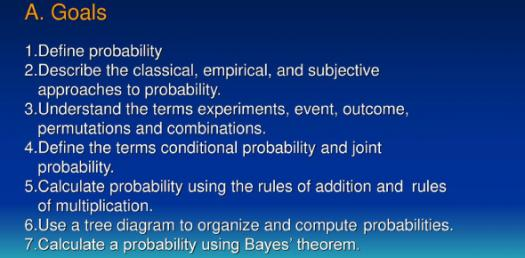# Quiz: Basic Probability Concept Questions!

16 Questions | Attempts: 238SettingsDo you know how to manage probability? To discover the probability, divide the possible number of events to occur by the total number of events. In contrast, the impossibility of the event can be discovered by subtracting the probability by one. Identify the number of possible outcomes for any event, and all potential outcomes must be identified first. Take this great test and find out what you know about probability.

• 1.
A drawer contains 3 red paperclips, 4 green paperclips, and 5 blue paperclips.  One paperclip is taken from the drawer and then replaced.  Another paperclip is taken from the drawer.  What is the probability that the first paperclip is red and the second paperclip is blue?
• 2.
A bag contains 8 green balls and 5 red balls.  Three balls are drawn one after another without replacing the balls drawn.  Find the probability of all the three balls drawn being green.
• 3.
In a taste test, 60 participants are randomly given a beverage to sample.  There are 15 samples of Pepsi, 15 samples of Coca Cola, and 10 samples of Sam's Choice and 20 samples of Dr. Thunder.  What is the probability of a participant not getting Sam's Choice Cola?
• 4.
If a point inside the figure is chosen at random, what is the probability that the point is inside the shaded region?
• 5.
When you roll a pair of dice, how many outcomes are there?
• 6.
There are 20 marbles in a bag, 4 blue, 4 green, 4 red, 4 white and 4 black.  Remember that all fraction answers should be given in the simplest form. What is the probability of drawing a black or white marble?
• A.

2/3

• B.

8/12

• C.

8/20

• D.

2/5

• 7.
If a month is picked at random, what is the probability that the month will start with M?
• A.

1/12

• B.

1/6

• C.

2/6

• D.

4/12

• 8.
On a game show, the game that a contestant plays is chosen by spinning a spinner.  The spinner has 5 colors on it:  red, green, blue, purple, and orange.   What is the complement of spinning a red or orange on this spinner? (Remember: complement = NOT)
• A.

Purple

• B.

Red, green, purple, orange, blue

• C.

Green, purple, blue

• 9.
Events are independent if the occurrence of one event does not affect the probability of the other.
• A.

True

• B.

False

• 10.
If P(A) > P(B), then event A is more likely to occur then event B.
• A.

True

• B.

False

• 11.
Which of the following formulas is used to find the probability of rolling a 2 or landing on tails?
• A.

P(A) x P(B)

• B.

P(A) + P(B)

• C.

P(A) x P(B/A)

• D.

P(B/A)

• 12.
Is [your statement here] true or false?
• A.

True

• B.

False

• 13.
Is the statement  "the larger sample size or more often you do an experiment, the closer your results will represent the entire population or  predicted theoretical number" true or false?
• A.

True

• B.

False

• 14.
In a group of 100 people, 19 were drinking punch and 23 were eating fish.  Find the probability that a person picked from this group at random is either eating fish or drinking punch.

## Related TopicsBack to top
×

Wait!
Here's an interesting quiz for you.